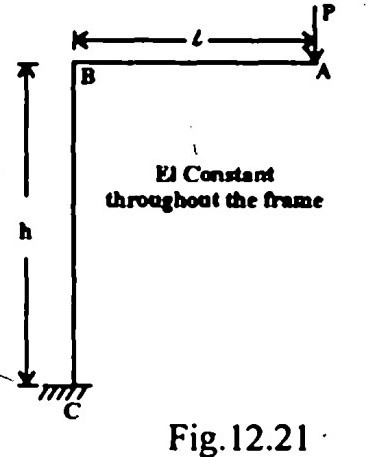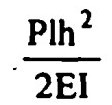Search

# Structural Analysis (81-100)

Updated: Apr 10, 2020

81. A force P is applied at the support B of the model (scale 1/10) of a two hinged arch as shown in Fig. 12.20. This force causes B to move through 100 mm and raises the crown C of the arch by 50 mm.In the prototype structure if a load of 10kN is applied at the crown, the horizontal

thrust produced will be 5 KN

82. A fixed beam of span L is carrying a point load P at its midspan. If the moment of interia of the middle half length is two times that of the remaining length, then

the fixed end moments will be 5PL/48

83.A fixed beam AB of span L is subjected to a clockwise moment M at a distance 'a' from end A. Fixed end moment at end A will be84Conjugate beam can be used to determine slopes and deflection in a non-prismatic beam,Conjugate beam method gives absolute slope and deflection

85. In a three hinged arch, maximum horizontal thrust occurs when the unit load is at the crown ,maximum sagging moment occurs when the unit load is at the section itself.

86. Influence line for horizontal thrust in a two hinged parabolic arch is cubic

87. For a single point load W moving on a symmetrical three hinged parabolic arch of span L, the maximum sagging moment occurs at a distance x from ends.

The value of x is 0.211 L

88. Muller Breslau's principle for obtaining influence lines is applicable to

i)trusses

ii) statically determinate beams and frames

iii) statically indeterminate structures, the material of which is elastic and

follows Hooke's law

89. Consider the, following statements in regard to finding stresses in truss members due to moving loads.

The stress in a web member is given by the influence line of shear force

for the panel containing the member,The stress in a chord member is given

by the influence line of bending moment for the node point opposite to

the member

90. In which of the following truss members the stress depends upon, whether the load is moving on top chord or bottom chord. verticals

91. For approximate analysis of building frames under vertical loads, the point of

inflection is assumed at one-tenth of the span length from each

end of the beam

92. In the cantilever method of lateral load analysis, which of the following assumptions are made ?

The intensity of axial stress in each,column of a storey is proportional to

the horizontal distance of that column from the centre of gravity of

all columns of the storey under consideration

93. The factor method of analysing building frames is

i) more accurate than either the portal or the cantilever method,

ii) based upon the slope-deflection method of analysis

94.A rigid cantilever frame ABC is fixed at C, as shown in Fig. 12.21. It carries a

point load P at A. Neglecting the axial deformation, the horizontal deflection AHA

of the point A will be95.1. The individual members are straight.

2. The individual members are connected by frictionless hinges.

3. The loads and reactions act only at the joints

96.Two bars AO and BO are of uniform area 'A' each and are hinged at O as shown in Fig. 12.22. A load W is applied at O.Wl/EI

97.The influence line for bending moment at section X (Mx), at a distance of 4m from the left support of a simply supported girder AB is shown in Fig. 12.23. A

uniformly distributed load of intensity 2t/m longer than the span crosses the girder from left to right.girder AB is shown in Fig. 12.23. A uniformly distributed load of intensity 2t/m longer than the span crosses the girder from left to right.48 tm

98.A one-storeyed rigid portal frame, ABCD, carries loads P and Q as shown in Fig.

12.24. It is hinged at the two supports A and D.indeterminate to the first degree

99.The influence line for shear at section X(FX) at a distance of 4 m from the left

support of a simply-supported girder AB is shown in Fig. 12.25.The shear force at section X due to a uniformly distributed dead load of

intensity t/m covering the entire span will be

8t

100.Consider the propped cantilever shown in Fig. 12.26 and the fixed beam shown in Fig. 12.27.The FEM at A of the fixed beam is 6 tm